Question

When a 23.8 mL sample of a 0.491 M aqueous acetic acid solution is titrated with a 0.381 M aqueous potassium hydroxide solution,

(1) What is the pH at the midpoint in the titration?

(2) What is the pH at the equivalence point of the titration?

(3) What is the pH after 46.0 mL of potassium hydroxide have been added

1)
At half equivalence point, pH = pKa

use:
pKa = -log Ka
= -log (1.8*10^-5)
= 4.7447

So, pH = 4.7447

2)
find the volume of KOH used to reach equivalence point
M(CH3COOH)*V(CH3COOH) =M(KOH)*V(KOH)
0.491 M *23.8 mL = 0.381M *V(KOH)
V(KOH) = 30.6714 mL
Given:
M(CH3COOH) = 0.491 M
V(CH3COOH) = 23.8 mL
M(KOH) = 0.381 M
V(KOH) = 30.6714 mL

mol(CH3COOH) = M(CH3COOH) * V(CH3COOH)
mol(CH3COOH) = 0.491 M * 23.8 mL = 11.6858 mmol

mol(KOH) = M(KOH) * V(KOH)
mol(KOH) = 0.381 M * 30.6714 mL = 11.6858 mmol

We have:
mol(CH3COOH) = 11.6858 mmol
mol(KOH) = 11.6858 mmol

11.6858 mmol of both will react to form CH3COO- and H2O

CH3COO- here is strong base
CH3COO- formed = 11.6858 mmol
Volume of Solution = 23.8 + 30.6714 = 54.4714 mL
Kb of CH3COO- = Kw/Ka = 1*10^-14/1.8*10^-5 = 5.556*10^-10
concentration ofCH3COO-,c = 11.6858 mmol/54.4714 mL = 0.2145M

CH3COO- dissociates as

CH3COO-        + H2O   ----->     CH3COOH +   OH-
0.2145                        0         0
0.2145-x                      x         x

Kb = [CH3COOH][OH-]/[CH3COO-]
Kb = x*x/(c-x)
Assuming x can be ignored as compared to c
So, above expression becomes

Kb = x*x/(c)
so, x = sqrt (Kb*c)
x = sqrt ((5.556*10^-10)*0.2145) = 1.092*10^-5

since c is much greater than x, our assumption is correct
so, x = 1.092*10^-5 M

[OH-] = x = 1.092*10^-5 M

use:
pOH = -log [OH-]
= -log (1.092*10^-5)
= 4.9619

use:
PH = 14 - pOH
= 14 - 4.9619
= 9.0381

3)
Given:
M(CH3COOH) = 0.491 M
V(CH3COOH) = 23.8 mL
M(KOH) = 0.381 M
V(KOH) = 46 mL

mol(CH3COOH) = M(CH3COOH) * V(CH3COOH)
mol(CH3COOH) = 0.491 M * 23.8 mL = 11.6858 mmol

mol(KOH) = M(KOH) * V(KOH)
mol(KOH) = 0.381 M * 46 mL = 17.526 mmol

We have:
mol(CH3COOH) = 11.6858 mmol
mol(KOH) = 17.526 mmol

11.6858 mmol of both will react

excess KOH remaining = 5.8402 mmol
Volume of Solution = 23.8 + 46 = 69.8 mL
[OH-] = 5.8402 mmol/69.8 mL = 0.0837 M

use:
pOH = -log [OH-]
= -log (8.367*10^-2)
= 1.0774

use:
PH = 14 - pOH
= 14 - 1.0774
= 12.9226

#### Earn Coins

Coins can be redeemed for fabulous gifts.

Similar Homework Help Questions
• ### When a 23.8 mL sample of a 0.443 M aqueous hydrofluorie acid solution is titrated with...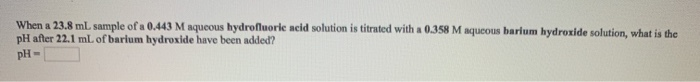When a 23.8 mL sample of a 0.443 M aqueous hydrofluorie acid solution is titrated with a 0.358 M aqueous barium hydroxide solution, what is the pH after 22.1 mL of barium hydroxide have been added? pH- What is the pH at the equivalence point in the titration of a 19.7 mL sample of a 0.376 M aqueous nitrous acid solution with a 0.447 M aqueous sodium hydroxide solution? pH- When a 20.1 mL sample of a 0.417 M aqueous...

• ### When a 19.8 mL sample of a 0.460 M aqueous acetic acid solution is titrated with...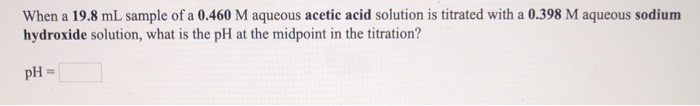When a 19.8 mL sample of a 0.460 M aqueous acetic acid solution is titrated with a 0.398 M aqueous sodium hydroxide solution, what is the pH at the midpoint in the titration? pH = A 17.5 mL sample of a 0.430 M aqueous hydrofluoric acid solution is titrated with a 0.381 M aqueous potassium hydroxide solution. What is the pH at the start of the titration, before any potassium hydroxide has been added? pH-

• ### When a 29,8 mL sample of a 0.322 M aqueous acetic acid solution is titrated with...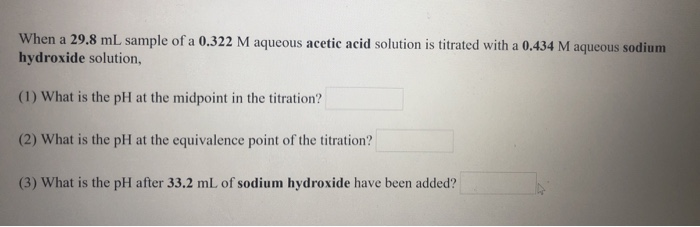When a 29,8 mL sample of a 0.322 M aqueous acetic acid solution is titrated with a 0,434 M aqueous sodium hydroxide solution, (1) What is the pH at the midpoint in the titration? (2) What is the pH at the equivalence point of the titration? (3) What is the pH after 33.2 mL of sodium hydroxide have been added?

• ### When a 23.2 mL sample of a 0.345 M aqueous acetic acid solution is titrated with...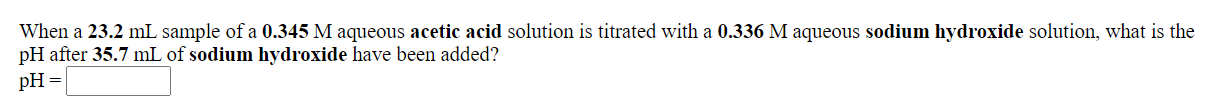When a 23.2 mL sample of a 0.345 M aqueous acetic acid solution is titrated with a 0.336 M aqueous sodium hydroxide solution, what is the pH after 35.7 mL of sodium hydroxide have been added? pH = What is the pH at the equivalence point in the titration of a 27.6 mL sample of a 0.423 M aqueous hydrocyanic acid solution with a 0.431 M aqueous potassium hydroxide solution? pH=

• ### When a 21.4 mL sample of a 0.311 M aqueous hydrocyanic acid solution is titrated with...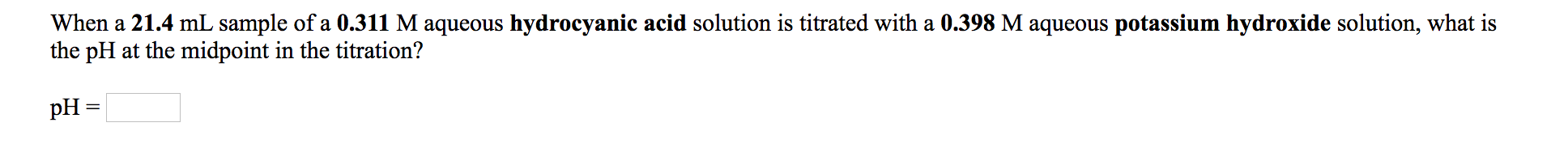When a 21.4 mL sample of a 0.311 M aqueous hydrocyanic acid solution is titrated with a 0.398 M aqueous potassium hydroxide solution, what is the pH at the midpoint in the titration? pH = A 45.3 mL sample of a 0.370 M aqueous acetic acid solution is titrated with a 0.424 M aqueous potassium hydroxide solution. What is the pH after 23.1 mL of base have been added? pH =

• ### 1) A 34.2 mL sample of a 0.590 M aqueous acetic acid solution is titrated with...

1) A 34.2 mL sample of a 0.590 M aqueous acetic acid solution is titrated with a 0.203 M aqueous potassium hydroxide solution. What is the pH after 37.1 mL of base have been added? 2) What is the pH at the equivalence point in the titration of a 25.5 mL sample of a 0.351 M aqueous hypochlorous acid solution with a 0.433 M aqueous barium hydroxide solution?

• ### When a 24.9 mL sample of a 0.438 M aqueous acetic acid solution is titrated with...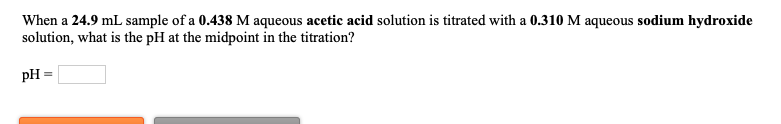When a 24.9 mL sample of a 0.438 M aqueous acetic acid solution is titrated with a 0.310 M aqueous sodium hydroxide solution, what is the pH at the midpoint in the titration? pH = What is the pH at the equivalence point in the titration of a 17.5 mL sample of a 0.380 M aqueous hydrofluoric acid solution with a 0.379 M aqueous barium hydroxide solution? PH = 3.14

• ### A 23.0 mL sample of a 0.497 M aqueous acetic acid solution is titrated with a...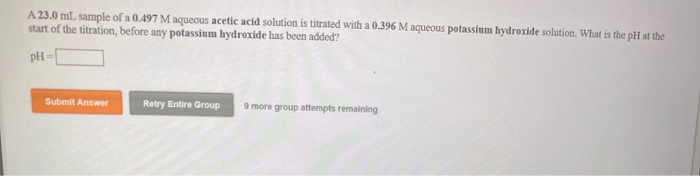A 23.0 mL sample of a 0.497 M aqueous acetic acid solution is titrated with a 0.396 M aqueous potassium hydroxide solution. What is the pH at the start of the titration, before any potassium hydroxide has been added? PH Submit Answer Retry Entire Group 9 more group attempts remaining What is the pH at the equivalence point in the titration of a 25.5 mL sample of a 0.411 M aqueous acetic acid solution with a 0.352 M aqueous barium...

• ### When a 17.2 mL sample of a 0.324 M aqueous hypochlorous acid solution is titrated with...

When a 17.2 mL sample of a 0.324 M aqueous hypochlorous acid solution is titrated with a 0.319 M aqueous barium hydroxide solution, (1) What is the pH at the midpoint in the titration? (2) What is the pH at the equivalence point of the titration? (3) What is the pH after 13.1 mL of barium hydroxide have been added?

• ### 1. When a 21.5 mL sample of a 0.329 M aqueous hypochlorous acid solution is titrated...

1. When a 21.5 mL sample of a 0.329 M aqueous hypochlorous acid solution is titrated with a 0.456 M aqueous potassium hydroxide solution, what is the pH after 23.3 mL of potassium hydroxide have been added? pH = 2. When a 16.0 mL sample of a 0.404 M aqueous hydrocyanic acid solution is titrated with a 0.418 M aqueous barium hydroxide solution, what is the pH at the midpoint in the titration? pH =